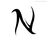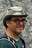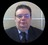Creating A invoice Number or Order Number

HI , i am trying to figure out how to automatically create a invoce number on each record Example INV0001,INV0002
as well as order number on each record SO0001, SO0002, same would go for item numbers ...

any help would be appreciated

Thank you.Hi, you can check the Invoices template for these calcs.

(Click New Database -> Invoices)

I did check it , However in the invoice template it works off the date and increments based on number

I waqs trying to add aincrementing number together with a text like "INV" without the date part.let c := cnt(select 'Invoices');
'Invoice No' := "INV-" + format(c, "000")format(c, "0000")

Thank you, Iwas not able to get it going

The furthest i got was with the below that gives me only the incremnting number for each invoice , but i couldnt get it to add the INV-000

let t := this; t.('Invoice Number' := max((select invoices).'Invoice Number') + 1)function left(sourceString : text,leftLen : number) do
substr(sourceString, 0, leftLen)
end;
function right(sourceString : text,rightLen : number) do
let len := length(sourceString);
let startPos := len - rightLen;
substr(sourceString, startPos, rightLen)
end;
let outputString := "00000000000000000000" + sourceString;
end;

so:
let t := this; t.('Invoice Number' := 'INV-' + pad(max((select invoices).'Invoice Number') + 1,4))

might be closer ...

let invNo:=max((select invoices).'Invoice Number';
let invNum:=right(invNo, length(invNo)-4);
let invNum:=invNum+1;

t.('Invoice Number' := 'INV-' + pad(invNum, 4)

is probably closer still ... not sure off the top of my head whether you will need to convert the text to number ... but hopefully this helps a bitmy pad function is probably over complicating, it was actually a part of something else I was doing in another process that I've just sort of stuck with ...

Try this:

let invNo:=max((select invoices).'Invoice Number';
let invNum:=right(invNo, length(invNo)-4);
let invNum:=invNum+1;

t.('Invoice Number' := format(invNum, "INV-0000"))

yes , after converting the field to text it started working , the problem was it was still a number field

Thank you all